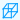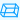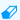Math Calculator

## Prism CalculatorTriangular Prism Area of Base :[ ½ab ] Perimeter of Base :[ s1 + s2 + s3 ] Surface Area of Prism :[ ab + (s1 + s2 + s3)h ] Volume of Prism :[ ½abh ] Enter the Altitude = Enter the Base = Enter the Height = Enter the Sides = Area of Base = Perimeter of Base = Surface Area of Prism = Volume of Prism =Rectangular Prism(Cuboid) Area of Base :[ lw ] Perimeter of Base :[ 2l + 2w ] Surface Area of Prism(Cuboid) :[ 2(lw) + (2l + 2w)h ] Volume of Prism(Cuboid) :[ lwh ] Diagonal of Prism(Cuboid) :[ Sqrt( l² + w² + h² ) ] Enter the Length = Enter the Width = Enter the Height = Area of Base = Perimeter of Base = Surface Area of Prism(Cuboid) = Volume of Prism(Cuboid) = Diagonal of Prism(Cuboid) =Regular Square Prism(Square Cuboid) Area of Base :[ s² ] Perimeter of Base :[ 4s ] Surface Area of Prism(Square Cuboid) :[ 2s² + 4sh ] Volume of Prism(Square Cuboid) :[ s²h ] Enter the Side = Enter the Height = Area of Base = Perimeter of Base = Surface Area of Prism(Square Cuboid) = Volume of Prism(Square Cuboid) =Regular Pentagonal Prism Area of Base :[ ½×a×5×s ] Perimeter of Base :[ 5s ] Surface Area of Prism :[ 5as + 5sh ] Volume of Prism :[ (5/2)ash ] Enter the Apothem Length= Enter the Side Length= Enter the Height = Area of Base = Perimeter of Base = Surface Area of Prism = Volume of Prism =Regular Hexagonal Prism Area of Base :[ ½×a×6×s ] Perimeter of Base :[ 6s ] Surface Area of Prism :[ 6as + 6sh ] Volume of Prism :[ 3ash ] Enter the Apothem Length= Enter the Side Length= Enter the Height = Area of Base = Perimeter of Base = Surface Area of Prism = Volume of Prism =